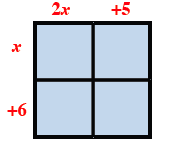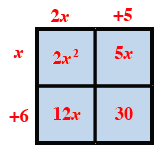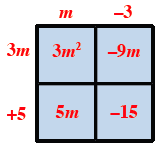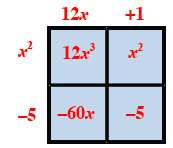### Home > CCA > Chapter 3 > Lesson 3.2.4 > Problem3-70

3-70.

Use a generic rectangle to multiply the following expressions. Write each solution both as a sum and as a product. Homework Help ✎

1. $(2x+5)(x+6)$

• Draw a generic rectangle and label the length and width.

• (2x + 5) (x + 6) = 2x2 +17x + 30

•• Fill in the grid by multiplying the length and width of each box.

• Write an equation showing the product is the sum of the parts.
Area as a sum: 2x2 + 12x + 5x + 30 = 2x2 + 17x + 30
Area as a product: (2x + 5)(x + 6)

1. $(m−3)(3m+5)$

• See the help for part (a).

•• Area as product: (m − 3)(3m + 5)
Area as sum: 3m2 − 4m − 15

1. $(12x+1)(x2−5)$

• See the help for part (a).

•1. $(3−5y)(2+y)$

• See the help for part (a).